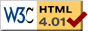### Journée-séminaire de combinatoire

#### (équipe CALIN du LIPN, université Paris-Nord, Villetaneuse)

Le 23 mai 2017 à 10h30 en B107, Hoang Ngoc Minh nous parlera de : Autour des associateurs

Résumé : This talk concerns the resolution of $KZ_3$ and our recent results on combinatorial aspects of zeta functions, $\{\zeta(s_1,\ldots,s_r)\}_{s_1,\ldots,s_r\in{\mathbb C}^r}$. In particular, we describe the action of the differential Galois group of $KZ_3$ on the asymptotic expansions of its solutions leading to a group of associators which contains the associator $\Phi_{KZ}$. Non trivial expressions of an associator with rational coefficients is also explicitly provided, based on the algebraic structures and the singularity analysis of the polylogarithms, $\{{\rm Li}_{s_1,\ldots,s_r}\}_{s_1,\ldots,s_r\in{\mathbb C}^r}$, and the harmonic sums, $\{H_{s_1,\ldots,s_r}\}_{s_1,\ldots,s_r\in{\mathbb C}^r}$.

 Dernière modification : Monday 24 January 2022Contact pour cette page : Cyril.Banderier at lipn.univ-paris13.fr